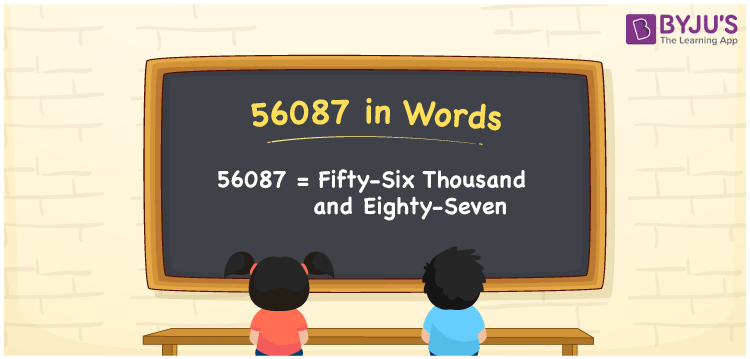# 56087 in words

56087 in words is written as Fifty Six Thousand and Eighty Seven. In 56087, 5 has a place value of ten thousand, 6 is in the place value of thousand, 0 is in the place value of hundred, 4 is in the place value of ten and 8 is in the place value of one. The article on Place Value gives more information. The number 56087 is used in expressions that relate to money, distance, length, social media views, and many more. For example, “My weekly total step count is Fifty Six Thousand and Eighty Seven.”

 56087 in words Fifty Six Thousand and Eighty Seven Fifty Six Thousand and Eighty Seven in Numbers 56087

## 56087 in English Words## How to Write 56087 in Words?

We can convert 56087 to words using a place value chart. This can be done as follows. The number 56087 has 5 digits, so let’s make a chart that shows the place value up to 5 digits.

 Ten thousand Thousands Hundreds Tens Ones 5 6 0 8 7

Thus, we can write the expanded form as:

5 × Ten thousand + 6 × Thousand + 0 × Hundred + 8 × Ten + 7 × One

= 5 × 10000 + 6 × 1000 + 0 × 100 + 8 × 10 + 7 × 1

= 56087.

= Fifty Six Thousand and Eighty Seven.

56087 is the natural number that is succeeded by 56086 and preceded by 56088.

56087 in words – Fifty Six Thousand and Eighty Seven.

Is 56087 an odd number? – Yes.

Is 56087 an even number? – No.

Is 56087 a perfect square number? – No.

Is 56087 a perfect cube number? – No.

Is 56087 a prime number? – Yes.

Is 56087 a composite number? – No.

## Solved Example

1. Write the number 56087 in expanded form

Solution: 5 x 10000 + 6 x 1000 + 0 x 100 + 8 x 10 + 7 x 1

We can write 56087 = 50000 + 6000 + 000 + 80 + 7

= 5 x 10000 + 6 x 1000 + 0 x 100 + 8 x 10 + 7 x 1.

## Frequently Asked Questions on 56087 in words

Q1

### How to write the number 56087 in words?

56087 in words is written as Fifty Six Thousand and Eighty Seven.
Q2

### Is 56087 divisible by 2?

No. 56087 is not divisible by 2.
Q3

### Is 56087 a prime number?

Yes. 56087 is a prime number.